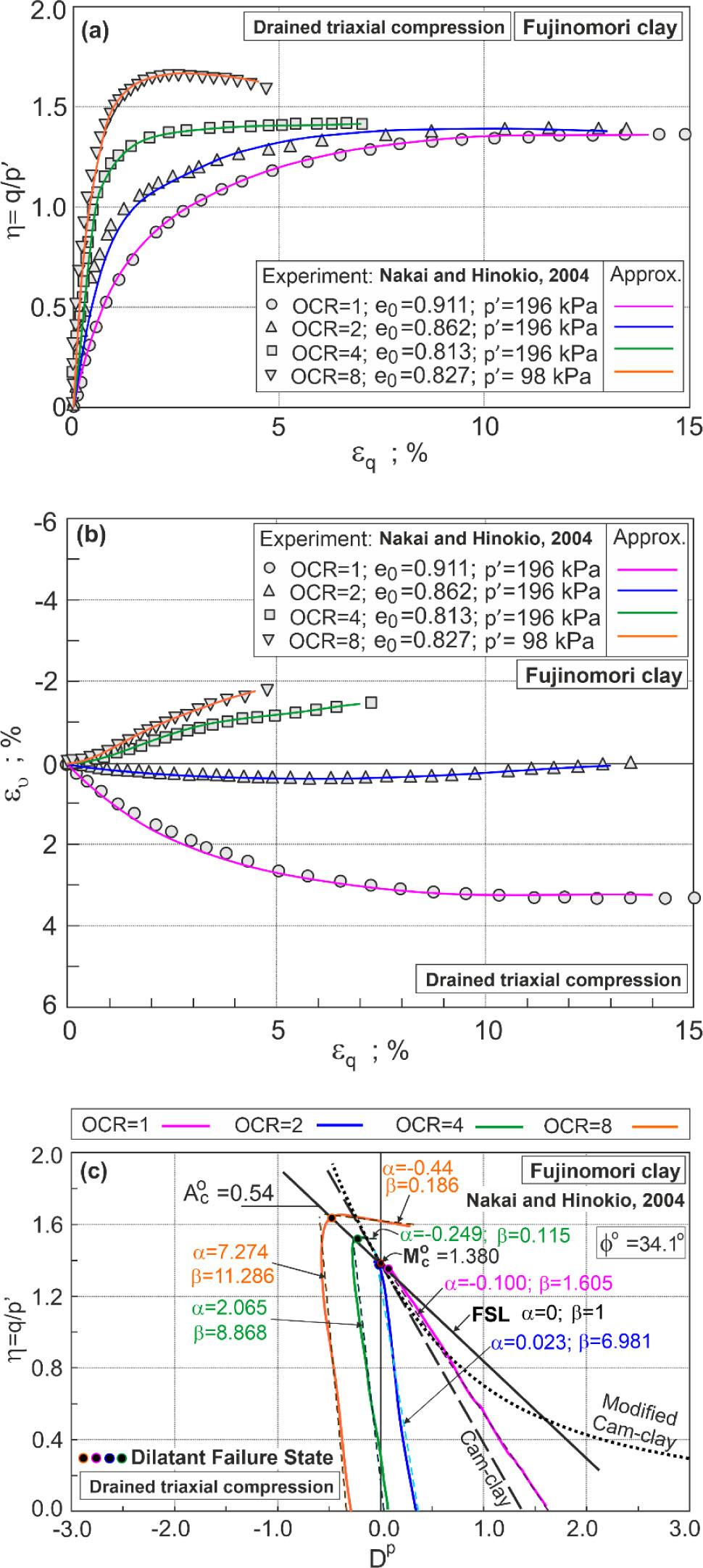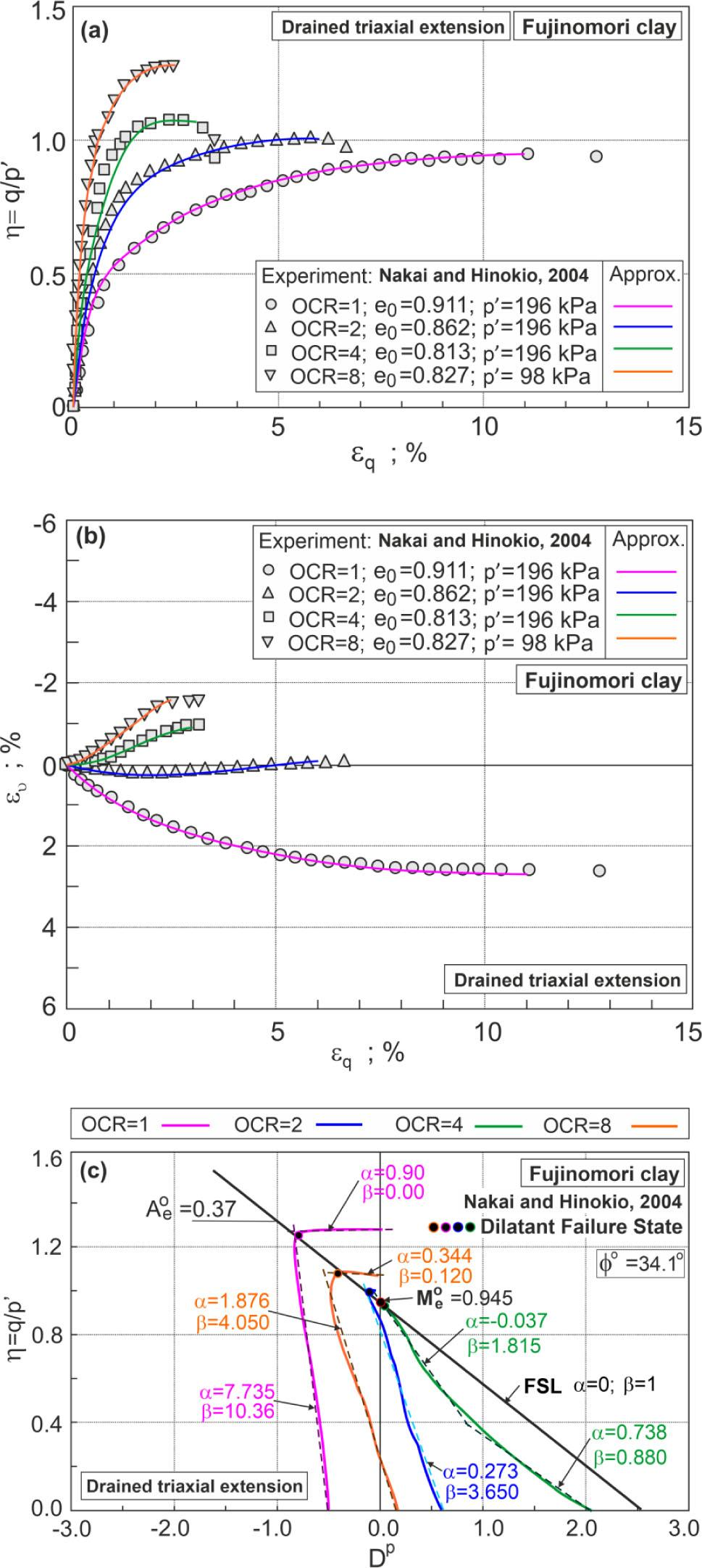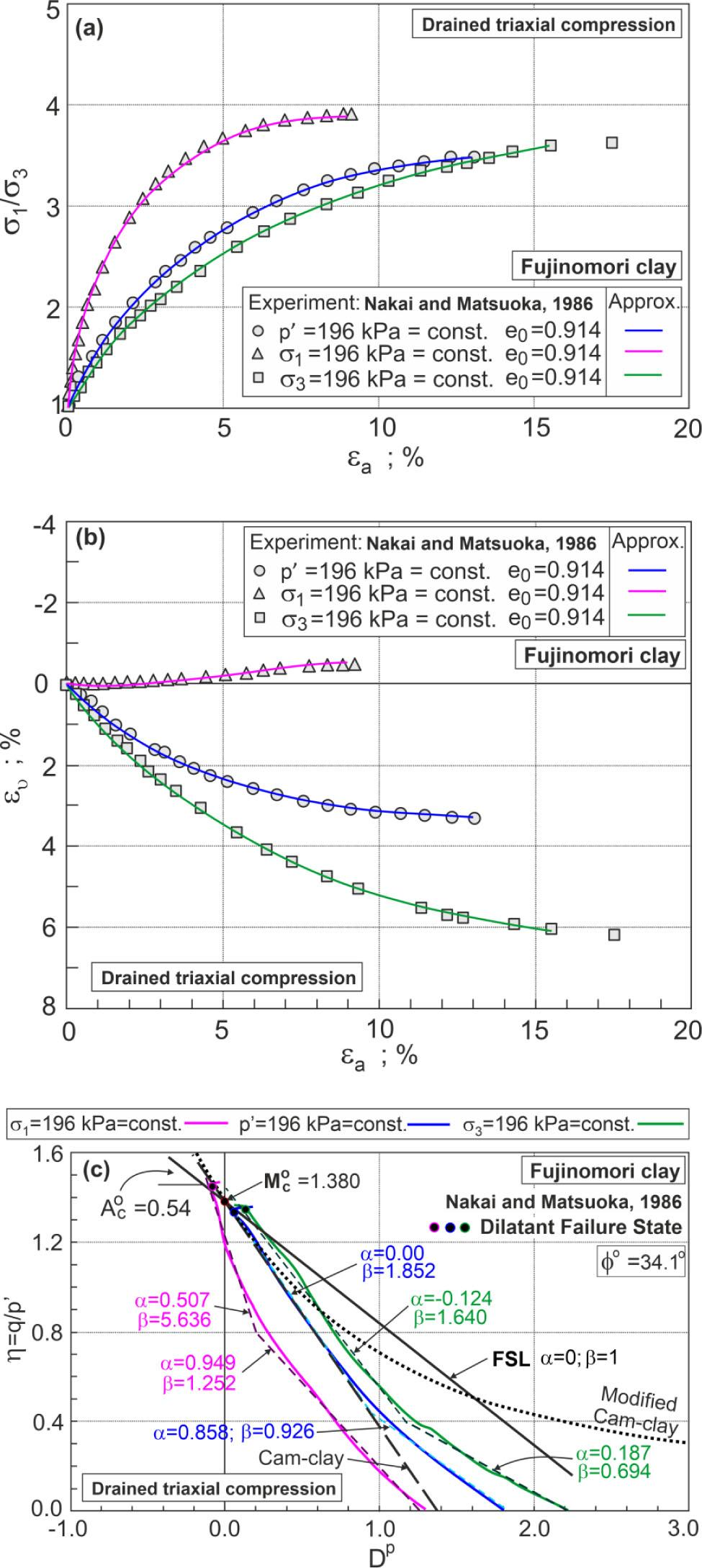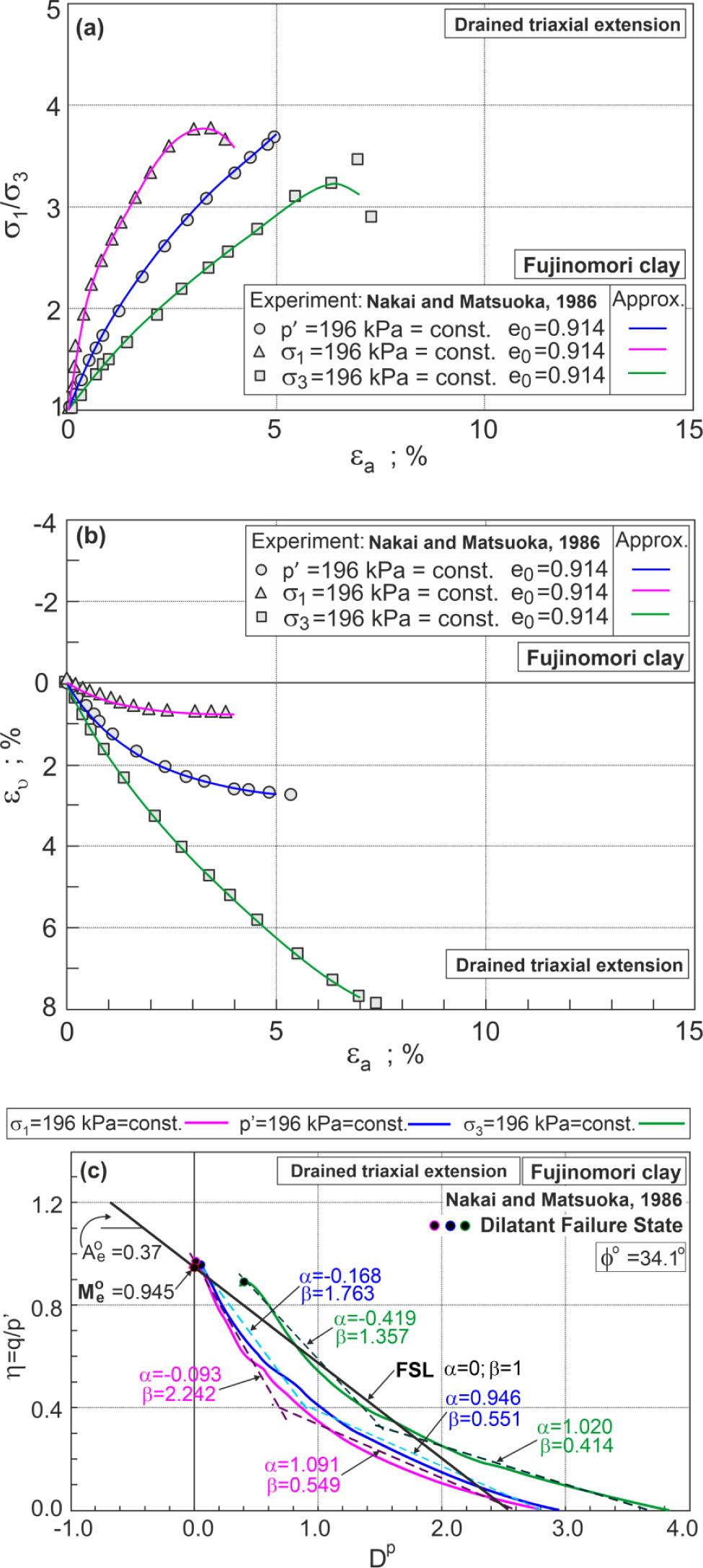Zeitschriftendaten
Format
Zeitschrift
eISSN
2083-831X
Erstveröffentlichung
09 Nov 2012
Erscheinungsweise
4 Hefte pro Jahr
Sprachen
Englisch
Uneingeschränkter Zugang

# Stress–dilatancy behaviour of remoulded Fujinomori clay

###### Seitenbereich: -
Zeitschriftendaten
Format
Zeitschrift
eISSN
2083-831X
Erstveröffentlichung
09 Nov 2012
Erscheinungsweise
4 Hefte pro Jahr
Sprachen
Englisch
Introduction

The phenomenon of soil dilatancy is the key to the characterising the strength and deformation of soils. The best known are the Rowe [10, 11] and Bolton  stress–dilatancy relationships for granular soils. Similarly, the dilatancy equations of Cam-clay and Modified Cam-clay  models are known for remoulded and normally consolidated clay. For structured and highly overconsolidated clays, however, the Cam-clay model’s stress–dilatancy relationships should be modified. By differentiating the plastic potential functions of the classical elasto-plastic model, various stress–plastic dilatancy relationships can be obtained . Szypcio  developed a general stress–plastic dilatancy relationship for soils based on the Frictional State Concept (FSC). The linear general stress–plastic dilatancy relationship defined by the critical frictional state angle (ϕo) and two (α, β) parameters of the soil can describe the real behaviour of soil at different stages of shearing. The Critical State Concept assumes that the critical frictional state angle is independent of the deformation mode (triaxial compression (TXC), plane strain and triaxial extension (TXE)), stress level, overconsolidation ratio (OCR) and the initial state of the soil. The critical state angle (ϕcs) for some soils depends on the deformation mode , and the stress level [5, 12] and structure of soil [3, 4]. The critical frictional state angle and the critical state angle are equal (ϕo=ϕcs) for sands and fully destructured clay .

In this paper, the stress–plastic dilatancy behaviour of Fujinomori clay during drained triaxial tests will be analysed. Data from drained TXC and TXE tests conducted by Nakai and Hinokio  with various OCRs and different stress paths conducted by Nakai and Matsuoka  will be taken for analysis. The influence of OCR and stress path on the stress–plastic dilatancy behaviour will be presented.

General dilatancy equation

The general stress ratio–plastic dilatancy equation of the FSC  is $η=Q−ADp$ \eta = Q - A{D^p} where $η=q/p′$ \eta = q/p' $Q=Mo−αAo$ Q = {M^o} - \alpha {A^o} $A=βAo$ A = \beta {A^o} $DP=δεvp/δεqp$ {D^P} = \delta \varepsilon _v^p/\delta \varepsilon _q^p where $δεvp=δεv−δεve$ \delta \varepsilon _v^p = \delta {\varepsilon _v} - \delta \varepsilon _v^e , $δεqp=δεq−δεqe$ \delta \varepsilon _q^p = \delta {\varepsilon _q} - \delta \varepsilon _q^e .

For drained TXC, $Mo=Mco=6sinϕo/3−sinϕo$ {M^o} = M_c^o = \left( {6{\rm{\;sin\;}}{\phi ^o}} \right)/\left( {3 - {\rm{\;sin\;}}{\phi ^o}} \right) $Ao=Aco=1−Mco/3$ {A^o} = A_c^o = 1 - M_c^o/3 $p′=σ1′+2σ3′/3$ p' = \left( {\sigma _1^\prime + 2\sigma _3^\prime} \right)/3 $δεv=δε1+2δε3$ \delta {\varepsilon _v} = \delta {\varepsilon _1} + 2\delta {\varepsilon _3} where σ1=σa is the conventional axial symmetry stress and σ1=σr=σθ are the conventional radial and circumferential stresses.

For drained TXE, $Mo=Meo=6sinϕo/3+sinϕo$ {M^o} = M_e^o = \left( {6{\rm{\;sin\;}}{\phi ^o}} \right)/\left( {3 + {\rm{\;sin\;}}{\phi ^o}} \right) $Ao=Aeo=1−2Meo/3$ {A^o} = A_e^o = 1 - 2M_e^o/3 $p′=2σ1′+σ3′/3$ p' = \left( {2\sigma _1^\prime + \sigma _3^\prime} \right)/3 $δεv=2δε1+δε3$ \delta {\varepsilon _v} = 2\delta {\varepsilon _1} + \delta {\varepsilon _3} where σ1=σr=σθ and σ3=σa are the conventional stresses for TXE.

For TXC and TXE, $q=σ1′−σ3′$ q = \sigma _1^\prime - \sigma _3^\prime $δεq=2δε1−δε3/3$ \delta {\varepsilon _q} = 2\left( {\delta {\varepsilon _1} - \delta {\varepsilon _3}} \right)/3 $δεvp=δεv−δεve$ \delta \varepsilon _v^p = \delta {\varepsilon _v} - \delta \varepsilon _v^e $δεqp=δεq−δεqe$ \delta \varepsilon _q^p = \delta {\varepsilon _q} - \delta \varepsilon _q^e $δεve=δp′/K$ \delta \varepsilon _v^e = \delta p'/K $δεqe=δq/3G$ \delta \varepsilon _q^e = \delta q/3G $K=v p′/κ$ K = v\;p'/\kappa $G=321−2ν1+νυp′κ$ G = {3 \over 2}{{1 - 2\nu } \over {1 + \nu }}{{\upsilon p'} \over \kappa } $v=1+e$ v = 1 + e where ϕo is the critical frictional state angle, e the void ratio, κ the slope of swelling line in the e-ln p’ plane (Cam-clay model parameter) and ν is the Poisson’s ratio.

For drained TXC, the dilatancy equation of the Cam-clay model is  $D=Mc−η$ D = {M_c} - \eta and of the Modified Cam-clay model is  $D=Mc2−η2/2η$ D = \left( {M_c^2 - {\eta ^2}} \right)/2\eta $Mc=6sinϕcs/3−sinϕcs$ {M_c} = 6{\rm{\;sin\;}}{\phi _{cs}}/\left( {3 - {\rm{\;sin\;}}{\phi _{cs}}} \right) where ϕcs is the critical state angle.

Tested soil

The results of drained triaxial tests of Fujinomori clay conducted by Nakai and Hinokio  and Nakai and Matsuoka  will be analysed. Specimens for tests were prepared by mixing natural Fujinomori clay (wL=44.7%, wP=24.7%, Gs=2.65) with deaerated water and were one dimensionally consolidated under the pressure of 49 kPa. The initial water content in the clay sample prepared in this way was approximately 40% [6, 8]. This method of sample preparation completely destroys the natural structure of Fujinomori clay.

The samples prepared in this way were consolidated isotropically to the pressure pc and unloaded to the pressure p0. In the experiments conducted by Nakai and Hinokio  for OCR = 1, 2, 4, p0=196 kPa and for OCR = 8, p0=98 kPa. In the drained TXC and TXE tests conducted by Nakai and Matsuoka , pc=p0=196 kPa (OCR = 1) and samples were loaded under different stress paths σ3=constant, p′=constant, σ1=constant. The void ratio of the clay sample at the onset of shear was calculated from the equation $e0=1.311−λ lnpc/49+κ lnpc/p0$ {e_0} = 1.311 - \lambda\, ln\left( {{p_c}/49} \right) + \kappa\, ln\left( {{p_c}/{p_0}} \right)

For Fujinomori clay, λ=0.105 and κ=0.023 [7, 8]. In the calculations, ν=0.15 was adopted.

Methodology

High-degree polynomials were used to segmentally approximate the relationships η-εq, ευ-εq (σ1/σ3-εa, ευ-εa) obtained in the tests. A lot of attention has been given to the connection conditions between the segments. At the connection points, there should not only be the same function values, but also a very small difference of the first derivatives. Approximation polynomials were used to calculate the stress–plastic dilatancy relationships. For the different stages of elasto-plastic deformation, the stress–plastic dilatancy relations were approximated by linear functions defined by Equation (1) and the parameters α and β were calculated for each stage. This procedure was described in detail in .

OCR effect of clay behaviour during shearing

The behaviour of the remoulded Fujinomori clay under different OCRs during drained TXC is shown in Figure 1. On the basis of the experimental results  of the relationship between the stress ratio and the shear strain (Fig. 1a) and of the volumetric strain and the shear strain (Fig. 1b), the relationship between the stress ratio and plastic dilatancy was calculated (Fig. 1c).Behaviour of Fujinomori clay under different OCRs in drained triaxial compression tests: a) stress ratio versus shear strain; b) volumetric strain versus shear strain; c) stress ratio versus plastic dilatancy.

The behaviour of clay during shear is highly dependent on OCR. For OCR = 1 and 2, contractive behaviour and for OCR = 4 and 8, dilative behaviour are observed. In all tests, plastic strains (Dp≠0) occur from the onset of the shear (Fig. 1c). Dilatant failure states (DFS) are defined as deformation states that are represented by the maximum curvature of the η-Dp curve. A similar definition of DFS was introduced in . These points are marked in Figure 1c. For the tested clay, DFS and failure states are different. The points representing DFS lie on the frictional state line (FSL) defined by Equation (1) with α=0 and β=1.0. According to FSC, FSL intersects the vertical axis at Moc= 1.38 (ϕo=34.1°), representing the slope of the critical state line in the q-p′ plane [13, 14]. The critical frictional state angle ϕo=34.1° is 0.4° higher than critical state angle ϕcs=33.7° [6, 8]. In the pre-DFS and post-DFS, the dependence of the stress ratio on plastic dilatancy can be approximated by straight lines defined by Equation (1) with different parameters α and β (Fig. 1c). The β parameter defining the slope of the approximated stress ratio–plastic dilatancy line in pre-DFS increases with OCR. For post-DFS, β≈0. For highly consolidated clay, the shear band is formulated in the sample and the calculated stresses and strains may not be completely correct. The stress–dilatancy relationships calculated from the equations of the Cam-clay model (23) and the Modified Cam-clay model (24) are also shown in Figure 1. The descriptions of the stress–dilatancy behaviour of remoulded clay normally consolidated under a constant mean pressure stress path with the use of FSC and Cam-clay models are very similar and correct. The Modified Cam-clay model badly describes the stress–dilatancy behaviour of the tested clay.

The behaviour of Fujinomori clay in the drained TXE tests is shown in Figure 2.Behaviour of Fujinomori clay under different OCRs in drained triaxial extension tests: a) stress ratio versus shear strain; b) volumetric strain versus shear strain; c) stress ratio versus plastic dilatancy.

As in the case of TXC, the relationships η-Dp in different shearing stages were approximated by straight lines defined by Equation (1) with different α and β parameters. The slope of these lines (β) increases with OCR. The points representing DFS lie almost exactly on the FSL defined by the critical frictional state angle ϕo=34.1°, α=0 and β=1. Fully contractive behaviour is observed only for normally consolidated (OCR = 1) clay and dilative behaviour is observed for lightly and heavily overconsolidated (OCR = 2, 4, 8) clay. The plastic dilatancy values for DFS are smaller than for TXC for the same initial conditions.

Influence of the stress path on the behaviour of clay during shearing

The influence of the stress path on the behaviour of remoulded Fujinomori clay in drained TXC and TXE was studied by Nakai and Matsuoka . The TXC and TXE tests were carried out for stress paths σ′3 - constant, p′ - constant and σ′1 - constant. The behaviour of Fujinomori clay is shown in Figure 3 and Figure 4 for TXC and TXE, respectively.Behaviour of Fujinomori clay in different stress paths for drained triaxial compression: a) principal stresses ratio versus axial strains; b) volumetric strains versus axial strains; c) stress ratio versus plastic dilatancy.Behaviour of Fujinomori clay in different stress paths for drained triaxial extension: a) principal stresses ratio versus axial strains; b) volumetric strains versus axial strains; c) stress ratio versus plastic dilatancy.

Linear approximations of the stress to plastic dilatancy ratio in different shearing stages are described by Equation (1) with the critical frictional state angle ϕo=34.1°, and the corresponding α and β parameters are shown in Figures 3c and 4c. In all tests conducted by Nakai and Matsuoka , two stages of shear behaviour can be identified in pre-DFS, unlike the tests conducted by Nakai and Hinokio  analysed earlier in this paper. DFS can be easily identified for all tests (Figs 3c and 4c). The points representing DFS for TXC lie very close to FSL (Fig. 3c), but not for TXE (Fig. 4c). Based on the presented analysis, it is impossible to finally conclude whether this is an error of the experiment or of the theory. For TXC, the η-Dp relationships of the Cam-clay and Modified Cam-clay models are also shown in Figure 3c. The dilatancy equation of the Cam-clay model (23) correctly describes the behaviour of remoulded, normally consolidated clay sheared under a constant mean pressure stress path. As expected, the stress path affects the shear behaviour of the clay. This effect can be quantified by α and β parameters of the general FSC dilatancy Equation (1).

Conclusions

OCR and stress path significantly influence the shearing behaviour of the remoulded clay.

The general dilatancy equation of FSC can be used to describe the behaviour of the stress–plastic dilatancy relationships of soils during shearing.

The Cam-clay model dilatancy equation describes well the stress–dilatancy behaviour only for remoulded, normally consolidated clay loaded under a constant mean pressure stress path.

DFS independent of OCR and stress paths are very characteristic states of soil behaviour during shear.

The points representing DFS lie on the friction state line defined in the η-Dp plane by the critical frictional state angle with parameters α=0 and β=1 for drained TXC and TXE.

The relationships between stress and plastic dilatancy, although rarely presented in the literature, are important for a full understanding of the behaviour of clay during shearing.

Balasubramaniam, A. S., Zue-Ming, H., Uddin, W., Chaudhry, A. R., Li, Y. G. (2007). Critical state parameters and peak stress envelopes for Bangkok clays. Q. J. Eng. Geol. Hydrogeol. 11(3), 219–232. https://doi.org/10.1144/GSL.QJEG.1978.011.03.02 BalasubramaniamA. S. Zue-MingH. UddinW. ChaudhryA. R. LiY. G. 2007 Critical state parameters and peak stress envelopes for Bangkok clays Q. J. Eng. Geol. Hydrogeol. 11 3 219 232 https://doi.org/10.1144/GSL.QJEG.1978.011.03.02 Search in Google Scholar

Bolton, M. D. (1986). The strength and dilatancy of sands. Géotechnique 36(1), 65–78. https://doi.org/10.1680/geot.1986.36.1.65 BoltonM. D. 1986 The strength and dilatancy of sands Géotechnique 36 1 65 78 https://doi.org/10.1680/geot.1986.36.1.65 Search in Google Scholar

Fearon, R. E. (1998). The behaviour of a structurally complex clay from an Italian landslide. PhD Dissertation, City University London, UK. https://openaccess.city.ac.uk/id/eprint/7575/ FearonR. E. 1998 The behaviour of a structurally complex clay from an Italian landslide PhD Dissertation, City University London UK https://openaccess.city.ac.uk/id/eprint/7575/ Search in Google Scholar

Fearon, R. E., Coop, M. R. (2000). Reconstitution: what makes an appropriate reference material? Géotechnique, 50(4), 471–477. https://doi.org/10.1680/geot.2000.50.4.471 FearonR. E. CoopM. R. 2000 Reconstitution: what makes an appropriate reference material? Géotechnique 50 4 471 477 https://doi.org/10.1680/geot.2000.50.4.471 Search in Google Scholar

Indraratna, B., Sun, Q. D., Nimbalkar, S. (2015). Observed and predicted behaviour of rail ballast under monotonic loading capturing particle breakage. Canadian Geotechnical Journal, 52(1), 73–86. https://doi.org/10.1139/cgj-2013-0361 IndraratnaB. SunQ. D. NimbalkarS. 2015 Observed and predicted behaviour of rail ballast under monotonic loading capturing particle breakage Canadian Geotechnical Journal, 52 1 73 86 https://doi.org/10.1139/cgj-2013-0361 Search in Google Scholar

Nakai, T., Matsuoka, H. (1986). A generalized elastoplastic constitutive model for clay in three-dimensional stresses. Soils and Foundations, 26(3), 81–98. https://doi.org/10.3208/sandf1972.26.3_81 NakaiT. MatsuokaH. 1986 A generalized elastoplastic constitutive model for clay in three-dimensional stresses Soils and Foundations 26 3 81 98 https://doi.org/10.3208/sandf1972.26.3_81 Search in Google Scholar

Nakai, T., Matsuoka, H., Okuno, N., Tsuzuki, K. (1986). True triaxial tests on normally consolidated clay and analysis of the observed shear behaviour using elastoplastic constitutive models. Soils and Foundations, 26(4), 67–78. https://doi.org/10.3208/sandf1972.26.4_67 NakaiT. MatsuokaH. OkunoN. TsuzukiK. 1986 True triaxial tests on normally consolidated clay and analysis of the observed shear behaviour using elastoplastic constitutive models Soils and Foundations 26 4 67 78 https://doi.org/10.3208/sandf1972.26.4_67 Search in Google Scholar

Nakai, T., Hinokio, M. A. (2004). A simple elastoplastic model for normally consolidated soils with unified material parameters. Soils and Foundations, 44(2), 53–70. https://doi.org/10.3208/sandf.44.2_53 NakaiT. HinokioM. A. 2004 A simple elastoplastic model for normally consolidated soils with unified material parameters Soils and Foundations 44 2 53 70 https://doi.org/10.3208/sandf.44.2_53 Search in Google Scholar

Rahimi, M. (2019). Review of Proposed Stress-Dilatancy Relationships and Plastic Potential Functions for Uncemented and Cemented Sands. J. Geol. Res. 1, 19–34. https://doi.org/10.30564/jgr.v1i2.864 RahimiM. 2019 Review of Proposed Stress-Dilatancy Relationships and Plastic Potential Functions for Uncemented and Cemented Sands J. Geol. Res. 1 19 34 https://doi.org/10.30564/jgr.v1i2.864 Search in Google Scholar

Rowe, P. W. (1962). The stress-dilatancy relation for static equilibrium of an assembly of particles in contacts. In Proceedings of the royal Society of London. Series A, Mathematical and Physical Sciences. 269(1339), 500–527. https://doi.org/10.1098/rspa.1962.0193 RoweP. W. 1962 The stress-dilatancy relation for static equilibrium of an assembly of particles in contacts In Proceedings of the royal Society of London. Series A, Mathematical and Physical Sciences 269 1339 500 527 https://doi.org/10.1098/rspa.1962.0193 Search in Google Scholar

Rowe, P. W. (1969). The relation between shear strength of sands in triaxial compression, plane strain and direct shear. Géotechnique, 19(1), 75–86. RoweP. W. 1969 The relation between shear strength of sands in triaxial compression, plane strain and direct shear Géotechnique 19 1 75 86 Search in Google Scholar

Shu, R., Kong, L., Liu, B., Wang, J. (2021). Stress-Strain Strength Characteristics of Undisturbed Granite Residual Soil Considering Different Patterns of Variation of Mean Effective Stress. Applied Sciences 11(4), 1874. https://doi.org/10.3390/app11041874 ShuR. KongL. LiuB. WangJ. 2021 Stress-Strain Strength Characteristics of Undisturbed Granite Residual Soil Considering Different Patterns of Variation of Mean Effective Stress Applied Sciences 11 4 1874 https://doi.org/10.3390/app11041874 Search in Google Scholar

Szypcio, Z. (2016). Stress-dilatancy for soils. Part I: The frictional state theory. Studia Geotechnica et Mechanica, 38(4), 51–57. https://doi.org/10.1515/sgem-2016-0030 SzypcioZ. 2016 Stress-dilatancy for soils. Part I: The frictional state theory Studia Geotechnica et Mechanica 38 4 51 57 https://doi.org/10.1515/sgem-2016-0030 Search in Google Scholar

Szypcio, Z. (2016). Stress-dilatancy for soils. Part II: Experimental validation for triaxial tests. Studia Geotechnica et Mechanica, 38(4), 59–65. https://doi.org/10.1515/sgem-2016-0031 SzypcioZ. 2016 Stress-dilatancy for soils. Part II: Experimental validation for triaxial tests Studia Geotechnica et Mechanica 38 4 59 65 https://doi.org/10.1515/sgem-2016-0031 Search in Google Scholar

Szypcio, Z., Dołżyk-Szypcio, K. (2022). The Stress-Dilatancy Behaviour of Artificially Bonded Soils. Materials, 15(20), 7068. https://doi.org/10.3390/ma15207068 SzypcioZ. Dołżyk-SzypcioK. 2022 The Stress-Dilatancy Behaviour of Artificially Bonded Soils Materials 15 20 7068 https://doi.org/10.3390/ma15207068 Search in Google Scholar

Wood, D. M. (1990). Shear behaviour and critical state soil mechanics. Cambridge University Press, New York, USA. WoodD. M. 1990 Shear behaviour and critical state soil mechanics Cambridge University Press New York, USA Search in Google Scholar

Empfohlene Artikel von Trend MD